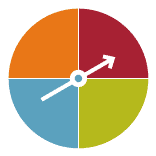#### Events

A six-sided die is rolled. We are interested in the probability of rolling an odd number. 'Rolling an odd number' is called an event. This event consists of one of three outcomes. These outcomes are 1, 3 or 5. The probability of rolling an odd number on a six-sided die when you roll just once is $$\dfrac{3}{6}$$ because there are three ways for an odd number to happen out of six equally likely outcomes.Detailed description

The probability of spinning red or green on a spinner divided into four regions as shown is $$\dfrac{2}{4}$$ because there are two ways for red or green to happen from four equally likely outcomes.